JEE  >  Test: Single Correct MCQs: Matrices and Determinants | JEE Advanced

# Test: Single Correct MCQs: Matrices and Determinants | JEE Advanced

Test Description

## 21 Questions MCQ Test Mathematics For JEE | Test: Single Correct MCQs: Matrices and Determinants | JEE Advanced

Test: Single Correct MCQs: Matrices and Determinants | JEE Advanced for JEE 2023 is part of Mathematics For JEE preparation. The Test: Single Correct MCQs: Matrices and Determinants | JEE Advanced questions and answers have been prepared according to the JEE exam syllabus.The Test: Single Correct MCQs: Matrices and Determinants | JEE Advanced MCQs are made for JEE 2023 Exam. Find important definitions, questions, notes, meanings, examples, exercises, MCQs and online tests for Test: Single Correct MCQs: Matrices and Determinants | JEE Advanced below.
Solutions of Test: Single Correct MCQs: Matrices and Determinants | JEE Advanced questions in English are available as part of our Mathematics For JEE for JEE & Test: Single Correct MCQs: Matrices and Determinants | JEE Advanced solutions in Hindi for Mathematics For JEE course. Download more important topics, notes, lectures and mock test series for JEE Exam by signing up for free. Attempt Test: Single Correct MCQs: Matrices and Determinants | JEE Advanced | 21 questions in 40 minutes | Mock test for JEE preparation | Free important questions MCQ to study Mathematics For JEE for JEE Exam | Download free PDF with solutions
 1 Crore+ students have signed up on EduRev. Have you?
Test: Single Correct MCQs: Matrices and Determinants | JEE Advanced - Question 1

### Consider the set  A of all determinants of order 3 with entries 0 or 1 only. Let B be the subset of A consisting of all determinants with value 1. Let C be the subset of A consisting of all determinants with value –1. Then

Detailed Solution for Test: Single Correct MCQs: Matrices and Determinants | JEE Advanced - Question 1

For every ‘det, with value 1’ (∈ B) we can find a det. with value – 1 by changing the sign of one entry of ‘1’.
Hence there are equal number of elements in B and C.
∴ (b) is the correct option

Test: Single Correct MCQs: Matrices and Determinants | JEE Advanced - Question 2

### If ω (≠1) is a cube root of unity, then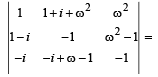Detailed Solution for Test: Single Correct MCQs: Matrices and Determinants | JEE Advanced - Question 2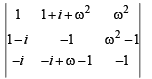Operating R1→ R1 – R2 + R3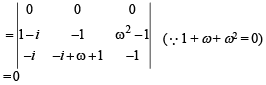Test: Single Correct MCQs: Matrices and Determinants | JEE Advanced - Question 3

### Let a, b, c be the real numbers. Then following system of equations in x, y and z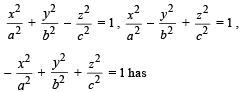Detailed Solution for Test: Single Correct MCQs: Matrices and Determinants | JEE Advanced - Question 3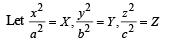Then the given system of equations becomes
X + Y – Z = 1
X – Y + Z= 1, – X + Y + Z = 1
This is the new system of equations For new system, we have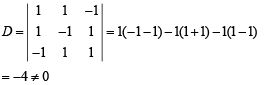∴ New system of equations has unique solution.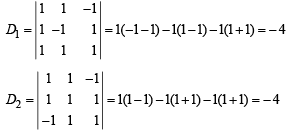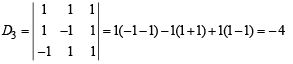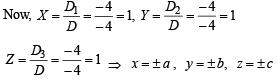Test: Single Correct MCQs: Matrices and Determinants | JEE Advanced - Question 4

If A and B are square matrices of equal degree, then which one is correct among the followings?

Detailed Solution for Test: Single Correct MCQs: Matrices and Determinants | JEE Advanced - Question 4

If A and B are square matrices of same degree then matrices A and B can be added or subtracted or multiplied. By algebra of matrices the only correct option is A + B = B + A

Test: Single Correct MCQs: Matrices and Determinants | JEE Advanced - Question 5

The parameter, on which the value of the determinant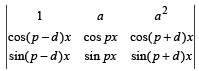does not depend upon is

Detailed Solution for Test: Single Correct MCQs: Matrices and Determinants | JEE Advanced - Question 5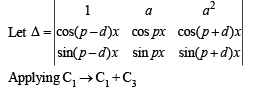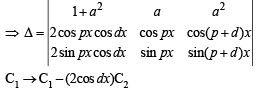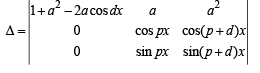Expanding along C1, we get
Δ = (1 + a2 – 2a cos dx) [sin (p + d) x cos px  – sin px cos (p + d)x]
⇒ Δ = (1 + a2 – 2a cos dx) [sin {(p + d)x – px}]
⇒ Δ = (1 + a2 – 2a cos dx) [sin dx]
which is independent of p.

Test: Single Correct MCQs: Matrices and Determinants | JEE Advanced - Question 6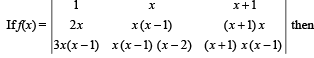f (100) is equal to

Detailed Solution for Test: Single Correct MCQs: Matrices and Determinants | JEE Advanced - Question 6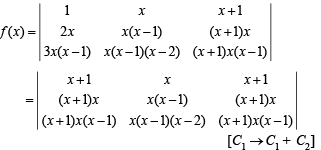= 0 [∵ C1 and C2 are identical] which is free of x, so the function is true for all values of x. Therefore, at x = 100, f (x) = 0, i.e., f (100) = 0

Test: Single Correct MCQs: Matrices and Determinants | JEE Advanced - Question 7

If the system of equations x – ky – z = 0, kx – y – z = 0, x + y – z = 0 has a non-zero solution, then the possible values of k are

Detailed Solution for Test: Single Correct MCQs: Matrices and Determinants | JEE Advanced - Question 7

For the given homogeneous system to have non zero solution determinant of coefficient matrix should be zero; i.e.,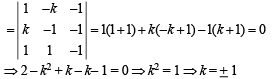Test: Single Correct MCQs: Matrices and Determinants | JEE Advanced - Question 8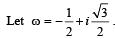Then the value of the determinant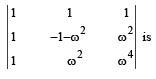Detailed Solution for Test: Single Correct MCQs: Matrices and Determinants | JEE Advanced - Question 8

Given that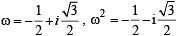Also 1 + ω + ω2 = 0 and ω3 = 1
Now given det. is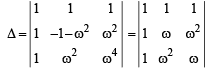[Using ω = –1 – ω2 and ω3 = 1]

Operating C1 → C1 + C2 + C3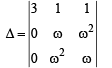(as 1 + ω + ω2 = 0)

Expanding along C1, we get
3 (ω2 – ω4) =3 (ω2 – ω) = 3ω (ω– 1)

Test: Single Correct MCQs: Matrices and Determinants | JEE Advanced - Question 9

The number of values of k for which the system of equations (k + 1)x + 8y = 4k;  kx + (k + 3) y = 3k – 1 has infinitely many solutions is

Detailed Solution for Test: Single Correct MCQs: Matrices and Determinants | JEE Advanced - Question 9

For infinitely many solutions the two equations become identical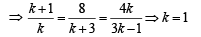Test: Single Correct MCQs: Matrices and Determinants | JEE Advanced - Question 10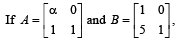hen value of α for which A2 = B, is

Detailed Solution for Test: Single Correct MCQs: Matrices and Determinants | JEE Advanced - Question 10

Given that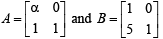and A2 = B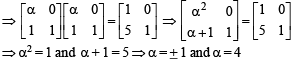∵ There is no common value
∴ There is no real value of a for which A2 = B

Test: Single Correct MCQs: Matrices and Determinants | JEE Advanced - Question 11

If the system of equations x + ay = 0, az + y = 0 and ax + z = 0 has infinite solutions, then the value of a is

Detailed Solution for Test: Single Correct MCQs: Matrices and Determinants | JEE Advanced - Question 11

The given system is, x + ay = 0
az + y = 0
ax + z = 0

It is system of homogeneous equations therefore, it will have infinite many solutions if determinant of coefficient matrix is zero. i.e.,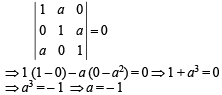Test: Single Correct MCQs: Matrices and Determinants | JEE Advanced - Question 12

Given  2x – y + 2z = 2, x – 2y + z = – 4, x + y + λz = 4 then the value of λ such that the given system of equation has NO solution, is

Detailed Solution for Test: Single Correct MCQs: Matrices and Determinants | JEE Advanced - Question 12

Since the system has no solution, Δ = 0 and any one amongst Dx, Dy, Dz is non-zero.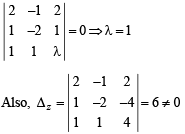Test: Single Correct MCQs: Matrices and Determinants | JEE Advanced - Question 13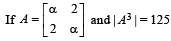then the value of α is

Detailed Solution for Test: Single Correct MCQs: Matrices and Determinants | JEE Advanced - Question 13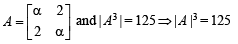Now, | A | = α2 – 4
⇒ (α2 – 4)3 = 125 = 53
⇒  α2 – 4 = 5
⇒  α = + 3

Test: Single Correct MCQs: Matrices and Determinants | JEE Advanced - Question 14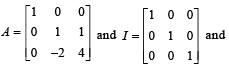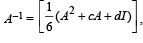then the value of c and d are

Detailed Solution for Test: Single Correct MCQs: Matrices and Determinants | JEE Advanced - Question 14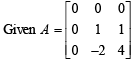∴ Characteristic eqn of above matrix A is given by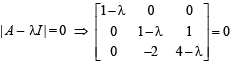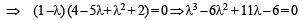Also by Cayley Hamilton thm (every square matrix satisfies its characteristic equation) we obtain
A3 – 6A2 + 11A – 6I = 0

Multiplying by A–1, we get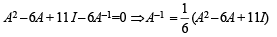Comparing it with given relation,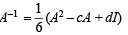we get c = – 6 and d = 11

Test: Single Correct MCQs: Matrices and Determinants | JEE Advanced - Question 15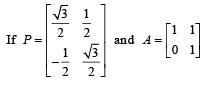and Q = PAPT and x = PTQ2005P then x is equal to

Detailed Solution for Test: Single Correct MCQs: Matrices and Determinants | JEE Advanced - Question 15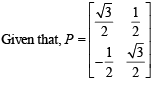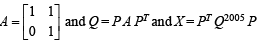We observe that Q = P A PT
⇒ Q2 = (P A PT) (P A PT)
= P A (PT P) A PT = PA (I A) PT
∴ P A2 PT
Proceeding in the same way, we get Q2005 = P A2005 PT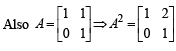and proceeding in the same way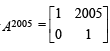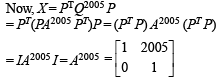Test: Single Correct MCQs: Matrices and Determinants | JEE Advanced - Question 16

Consider three points

P = (- sin(β - α), - cosβ), Q = (cos(β-α), sin β) and
R = (cos(β- α+θ), sin(β- θ)) , where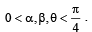Then,

Detailed Solution for Test: Single Correct MCQs: Matrices and Determinants | JEE Advanced - Question 16

The given points are P (- sin(β- α), – cosβ) , Q(cos(β - a), sinβ)
R(cos(β- α+θ), sin(β- θ))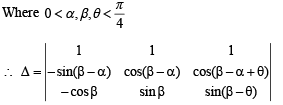Operating C3 - C1 sinθ- C2 cosθ , we get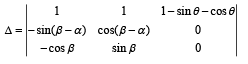= (1 - sinθ - cosθ)[cosβ cos(β -α) - sin β sin(β-α)]
⇒ Δ = [1 - (sinθ+ cosθ)] cos(2β-α)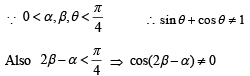∴ Δ≠ 0 ⇒ the three points are non collinear..

Test: Single Correct MCQs: Matrices and Determinants | JEE Advanced - Question 17

The number of 3 ×  3 matrices A whose entries are either 0 or1 and for which the system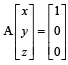has exactly twodistinct solutions, is

Detailed Solution for Test: Single Correct MCQs: Matrices and Determinants | JEE Advanced - Question 17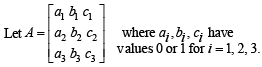Then the given system is equivalent to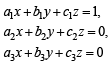Which represents three distinct planes. But three planes can not intersect at two distinct points, therefore no such system exists.

Test: Single Correct MCQs: Matrices and Determinants | JEE Advanced - Question 18

Let ω ≠ 1 be a cube root of unity and S be the set of all non-singular matrices of the form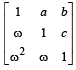where each of a, b and c is either ω or ω2. Then the number of distinct matrices in the set S is

Detailed Solution for Test: Single Correct MCQs: Matrices and Determinants | JEE Advanced - Question 18

For the given matrix to be non-singular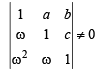⇒ 1 – (a + c) ω + a cω2 ≠ 0 ⇒ (1 – aω) (1 – cω) ≠ 0
⇒ a ≠ ω2 and c ≠ ω2 where ω is complex cube root of unity.
As a, b and c are complex cube roots of unity
∴ a and c can take only one value i.e. w while b can take 2 values i.e. ω and ω2. ∴ Total number of distinct matrices = 1 × 1 × 2 = 2

Test: Single Correct MCQs: Matrices and Determinants | JEE Advanced - Question 19

Let P = [aij] be a 3 x 3 matrix and let Q = [bij], where bij = 2i + j   aij for 1 < i , j < 3. If the determinant of P is 2, then the determinant of the matrix Q is

Detailed Solution for Test: Single Correct MCQs: Matrices and Determinants | JEE Advanced - Question 19

We have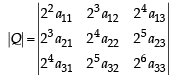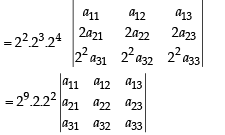= 212 x |P| = 212 x 2 = 213

Test: Single Correct MCQs: Matrices and Determinants | JEE Advanced - Question 20

If P is a 3 x 3 matrix such that PT = 2P + I, where PT is the transpose of P and I is the 3 x 3 identity matrix, then there
exists a column matrix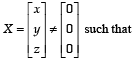Detailed Solution for Test: Single Correct MCQs: Matrices and Determinants | JEE Advanced - Question 20

We have PT = 2P + I
⇒ P = 2PT + I ⇒ P = 2(2P + I) + I
⇒ P = 4P + 3I ⇒ P + I = 0
⇒ PX + X = 0 ⇒ PX = – X

Test: Single Correct MCQs: Matrices and Determinants | JEE Advanced - Question 21

Let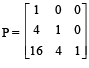and I be the identity matrix of order 3. If Q = [qij] is a m atrix such that P50 – Q = I, then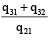equals

Detailed Solution for Test: Single Correct MCQs: Matrices and Determinants | JEE Advanced - Question 21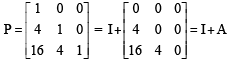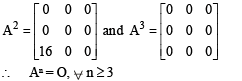Now P50 = (I + A)50 = 50C0 I50 + 50C1 I49 A + 50C2 I48 A2 + O
= I + 50A + 25 × 49 A2.
∴ Q = P50 – I = 50A + 25 × 49 A2.
⇒ q21 = 50 × 4 = 200
⇒ q31 = 50 × 16 + 25 × 49 × 16 = 20400
⇒ q32 = 50 × 4 = 200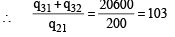## Mathematics For JEE

130 videos|359 docs|306 tests
 Use Code STAYHOME200 and get INR 200 additional OFF Use Coupon Code
Information about Test: Single Correct MCQs: Matrices and Determinants | JEE Advanced Page
In this test you can find the Exam questions for Test: Single Correct MCQs: Matrices and Determinants | JEE Advanced solved & explained in the simplest way possible. Besides giving Questions and answers for Test: Single Correct MCQs: Matrices and Determinants | JEE Advanced, EduRev gives you an ample number of Online tests for practice

## Mathematics For JEE

130 videos|359 docs|306 tests